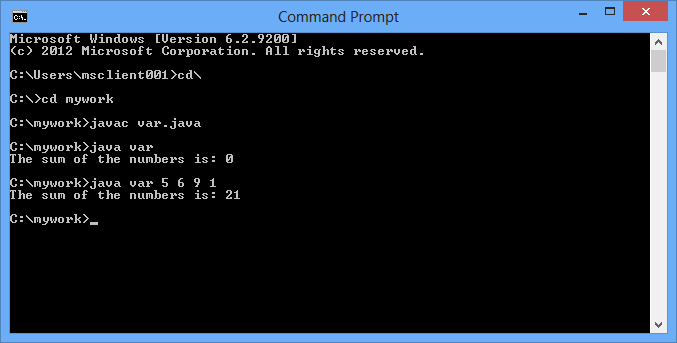##### What is Varargs in Java?

Varargs (variable arguments) is a feature introduced in Java 1.5. It allows a method take an arbitrary number of values as arguments.

##### How Varargs Work?

When varargs facility is used, it actually first creates an array whose size is the number of arguments passed at the call site, then puts the argument values into the array, and finally passes the array to the method.

##### When to Use Varargs?

As its definition indicates, varargs is useful when a method needs to deal with an arbitrary number of objects. One good example from Java SDK is String.format(String format, Object... args). The string can format any number of parameters, so varargs is used.

##### Following are the two example using Varargs :

1)    class sumvar

`{    public static void main(String[] args)    {        int result1 = sum(1, 2);        int result2 = sum(1, 2, 3);        int result3 = sum(1, 2, 4, 5, 6, 1000);         System.out.println(result1 + "\n" + result2 + "\n" + result3);    }    public static int sum(int... args)    {        int add = 0;         if (args != null)        {            for (int val : args)            {                add += val;            }        }        return add;    }}`

This will give the following output:2)    public class var

` {    public static void main(String args[])   {      var obj= new var();      String sum="";      sum = obj.add(args);      System.out.println("The sum of the numbers is: " + sum);   }    public static String add(String... args)   {      int sum, i;      sum=0;      for(i=0; i< args.length; i++)    {         sum += Integer.parseInt(args[i]);      }            String s= Integer.toString(sum);      return(s);      }}`

This will give the following output:The above two examples are different in the way that one of them passes values as the argument and other ask user to input value at run time.

Modified On Mar-13-2018 04:49:21 AM